# Analyzing Motion in Connected Rigid Bodies (Part - 3) Civil Engineering (CE) Notes | EduRev

## Civil Engineering (CE) : Analyzing Motion in Connected Rigid Bodies (Part - 3) Civil Engineering (CE) Notes | EduRev

The document Analyzing Motion in Connected Rigid Bodies (Part - 3) Civil Engineering (CE) Notes | EduRev is a part of the Civil Engineering (CE) Course Introduction to Dynamics and Vibrations- Notes, Videos, MCQs.
All you need of Civil Engineering (CE) at this link: Civil Engineering (CE)

6.3.3 Gears

Gears can be analyzed in much the same way as a rolling wheel. Gears are used to increase or decrease angular velocities (they act like mechanical amplifiers): for example, in the animation the small gear is rotating at twice the angular rate of the large one. They also modify the torques (or moments) applied to the gears: if a gear system increases angular velocity, it reduces torque by the same factor (so the torque on the small gear in the animation is half that on the large one). Some clever gear systems can even be used to add angular velocities (see the discussion of epicyclic gears below.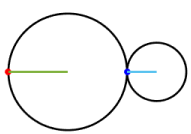There are many different gear designs.  Here, we focus only on two-dimensional ‘spur gears’. Spur gears have a rather complicated geometry, which we don’t have time to discuss in detail in this course.   They are designed to behave like two wheels which roll against each other with no slip at the contact. The wheel radius is equal to the ‘pitch circle radius’ of the gears (which is slightly smaller than physical diameter of the gears, because the teeth have to overlap). Gear manufacturers often specify the number of teeth on a gear instead of its radius. The number of teeth and the radius have to be related, because the teeth have to be the same circumferential distance apart for the gear pair to mesh.

We analyze motion of gears using two ideas:

1. Two meshed gears must have equal velocities at the point where they touch.
2. The rigid body formula, relating the velocity of points on the circumference of the gear to the velocity of its center:

v= vO + ωzk × (rC− rO)

In practice we don’t usually bother doing the cross product, and instead just write down the velocity on the circumference directly using the figure provided: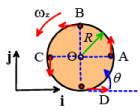• vA =vO + ωzRj
• vB =vO −ωzRi
• vC =vO − ωzRj
• vD =vO + ωzRi

You don’t have to remember these – just visualize every point on the gear moving in circular motion (counterclockwise) around O, and write down the vectors (be careful with signs!).

Example1: The left gear in the figure rotates with counterclockwise angular velocity ωz1. The large gear has radius R1 and N1 teeth, the small one has radius R2 and N2 teeth. Calculate the angular velocity of the smaller gear.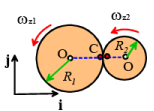Note:

• The velocities of the two touching gears are equal at C
• The gear rotation/velocity formula gives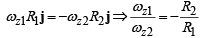Notice that we assume both gears rotate counterclockwise. The formula tells us that the second gear has a negative angular velocity – this means that it is actually rotating clockwise. The animation at the top of this section confirms that this indeed is the case.

Since the distance between two teeth is the same on each gear, the number of teeth must be proportional to the radius. This means we can also write the gear ratio in terms of N1 and N2

ωz1/ωz2 = -N2/N1

Example 2: An ‘epicyclic’ gearbox is a special arrangement of gears that has many applications. The sketch shows a simple example. The gearbox can be driven in three different places: one drive shaft is connected to the central sun gear (A); the other is attached to the ‘planet carrier’, which is joined to the center of the ‘pinion gears’ B,C and D. The outer gear (E – called the ‘ring gear’) can also be driven separately.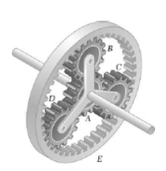Epicyclic gearboxes are used in all automatic vehicle transmissions. They are also very useful in ‘split power’ drives, where two motors need to be connected together to drive a single axle. Hybrid vehicles, which have both an electric motor and an internal combustion engine driving the same axle, are one example. You can find a very nice description of the Toyota Prius split power transmission here:  the website includes a Flash animation that lets you change the speeds of the motors in the system and visualize the motion of the gears.

The figure shows a schematic diagram illustrating the general geometry and motion of the system. We have four rigid bodies:

• The central sun gear, radius RS, NS teeth, rotating at angular velocity ωzS
• The planet carrier, angular velocity ωzPC
• The ring gear, radius RR, with NR teeth, angular velocity ωzR
• The planet gear, radius r = (RR − RS )/ 2 ,NP = ( NR −NS )/ 2 teeth, rotating at angular velocity ωzP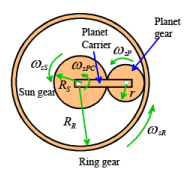In any application, we are given the angular velocity of two of the drive shafts (any two of ωzSzPCzR ), and must calculate the third. The planet gear is not connected to any drive shaft, so we usually don’t care very much about its angular speed, but we will need to find ωzP to solve for the unknown one of ωzSzPCzR

This seems a terribly difficult problem, but it can be solved in a very simple way with a trick.

We start by solving a simpler version of the problem.  Suppose that the planet carrier is stationary (ωzPC =0) and the sun gear rotates with angular speed  ωzS (see the animation).   What is the angular velocity of the ring gear?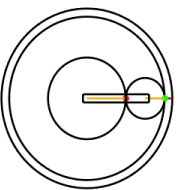The sun gear and the planet gear are just a standard gear pair so we know that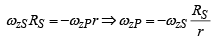The two touching points on the planet gear and the ring gear must have the same velocity, so (using the rotating gear formula)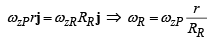We can eliminate ωzP to get the answer: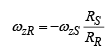Now let’s try the harder problem. The animation shows a general situation, where ωzSzPC are both nonzero. How can we find ωzR now?

This is difficult to analyze because the center of the planet gear is not fixed, so it’s hard for us to visualize the motion, and the standard gear formulas don’t work. But we can simplify the problem by analyzing motion in a reference frame that rotates with the planet carrier.   For example, imagine attaching a videocamera to the planet carrier – this camera would show the planet carrier to be stationary, with the surrounding world rotating in the opposite direction. The angular velocity of the planet carrier would be subtracted from all the other angular velocities. In this reference frame, we can use the result we just calculated: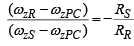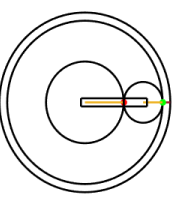This result is general, and can be re-arranged to tell you the angular velocities for any given combination of ωzSzPC and ωzR .

Offer running on EduRev: Apply code STAYHOME200 to get INR 200 off on our premium plan EduRev Infinity!

## Introduction to Dynamics and Vibrations- Notes, Videos, MCQs

20 videos|53 docs

,

,

,

,

,

,

,

,

,

,

,

,

,

,

,

,

,

,

,

,

,

;